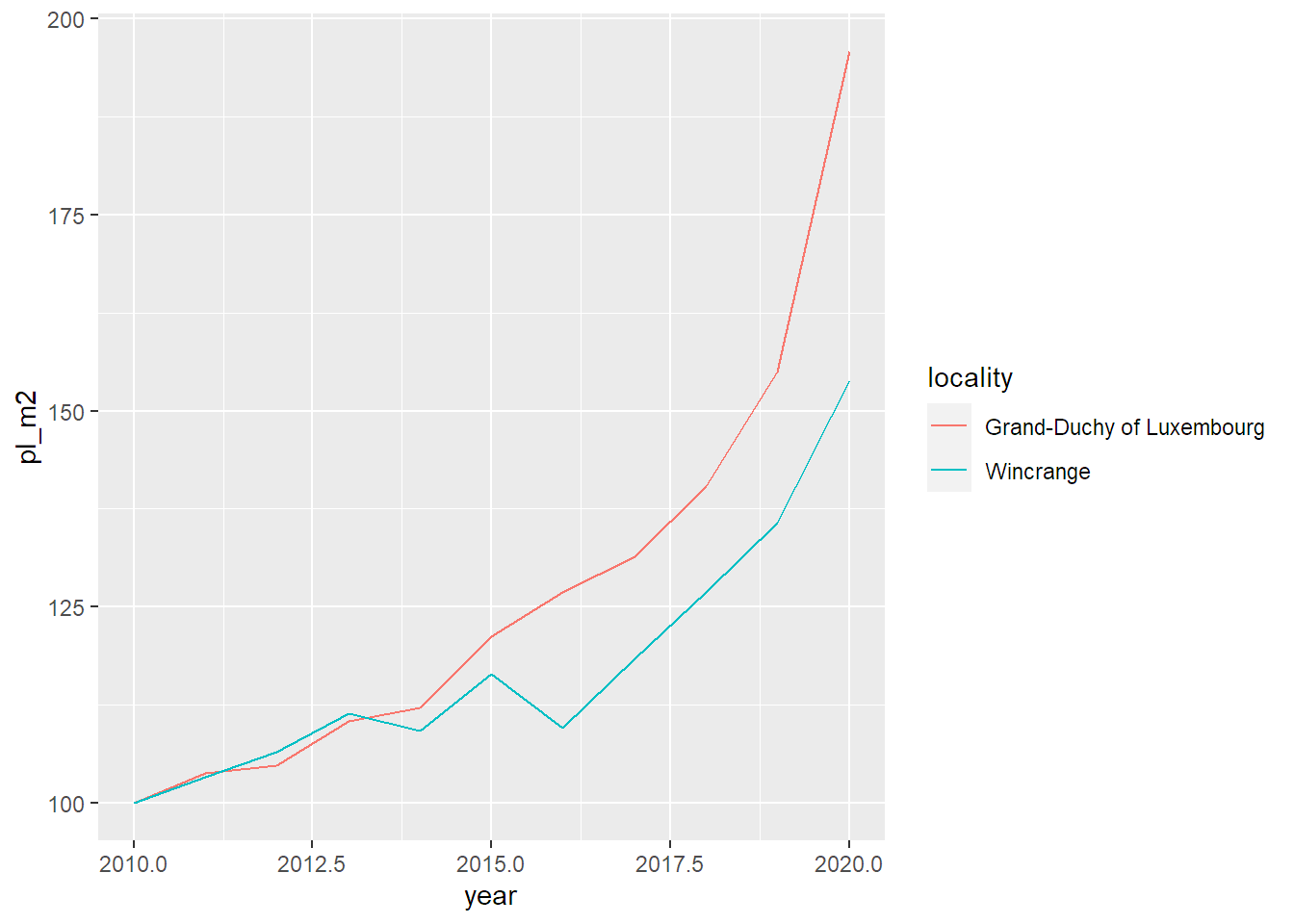library(dplyr)
##
## Attaching package: 'dplyr'
## The following objects are masked from 'package:stats':
##
##     filter, lag
## The following objects are masked from 'package:base':
##
##     intersect, setdiff, setequal, union
library(ggplot2)
library(purrr)
library(tidyr)

commune_level_data <- read.csv(
"../datasets/house_prices_commune_level_data.csv"
)

"../datasets/house_prices_country_level_data.csv"
)

Let’s compute the Laspeyeres index for each commune:

get_laspeyeres <- function(dataset){

which_dataset <- deparse(substitute(dataset))

group_var <- if(grepl("commune", which_dataset)){
quo(locality)
} else {
NULL
}
dataset %>%
group_by(!!group_var) %>%
mutate(p0 = ifelse(year == "2010", average_price_nominal_euros, NA)) %>%
fill(p0, .direction = "down") %>%
mutate(p0_m2 = ifelse(year == "2010", average_price_m2_nominal_euros, NA)) %>%
fill(p0_m2, .direction = "down") %>%
ungroup() %>%
mutate(pl = average_price_nominal_euros/p0*100,
pl_m2 = average_price_m2_nominal_euros/p0_m2*100)

}
commune_level_data <- get_laspeyeres(commune_level_data)
#commune_level_data <- commune_level_data %>%
#  group_by(locality) %>%
#  mutate(p0 = ifelse(year == "2010", average_price_nominal_euros, NA)) %>%
#  fill(p0, .direction = "down") %>%
#  mutate(p0_m2 = ifelse(year == "2010", average_price_m2_nominal_euros, NA)) %>%
#  fill(p0_m2, .direction = "down") %>%
#  ungroup() %>%
#  mutate(pl = average_price_nominal_euros/p0*100,
#         pl_m2 = average_price_m2_nominal_euros/p0_m2*100)

Let’s also compute it for the whole country:

country_level_data <- get_laspeyeres(country_level_data)
#country_level_data <- country_level_data %>%
#  mutate(p0 = ifelse(year == "2010", average_price_nominal_euros, NA)) %>%
#  fill(p0, .direction = "down") %>%
#  mutate(p0_m2 = ifelse(year == "2010", average_price_m2_nominal_euros, NA)) %>%
#  fill(p0_m2, .direction = "down") %>%
#  mutate(pl = average_price_nominal_euros/p0*100,
#         pl_m2 = average_price_m2_nominal_euros/p0_m2*100)

We are going to create a plot for 5 communes and compare the price evolution in the communes to the national price evolution. Let’s first list the communes:

communes <- c("Luxembourg",
"Esch-sur-Alzette",
"Mamer",
"Schengen",
"Wincrange")
make_plot <- function(commune){

commune_data <- commune_level_data %>%
filter(locality == commune)

data_to_plot <- bind_rows(
country_level_data,
commune_data
)

ggplot(data_to_plot) +
geom_line(aes(y = pl_m2,
x = year,
group = locality,
colour = locality))
}
res <- lapply(communes, function(x){

knitr::knit_child(text = c(

'\n',
'## Plot for commune: r x',
'\n',
'{r, echo = F}',
'print(make_plot(x))',
''

),
envir = environment(),
quiet = TRUE)

})

cat(unlist(res), sep = "\n")

## Plot for commune: Luxembourg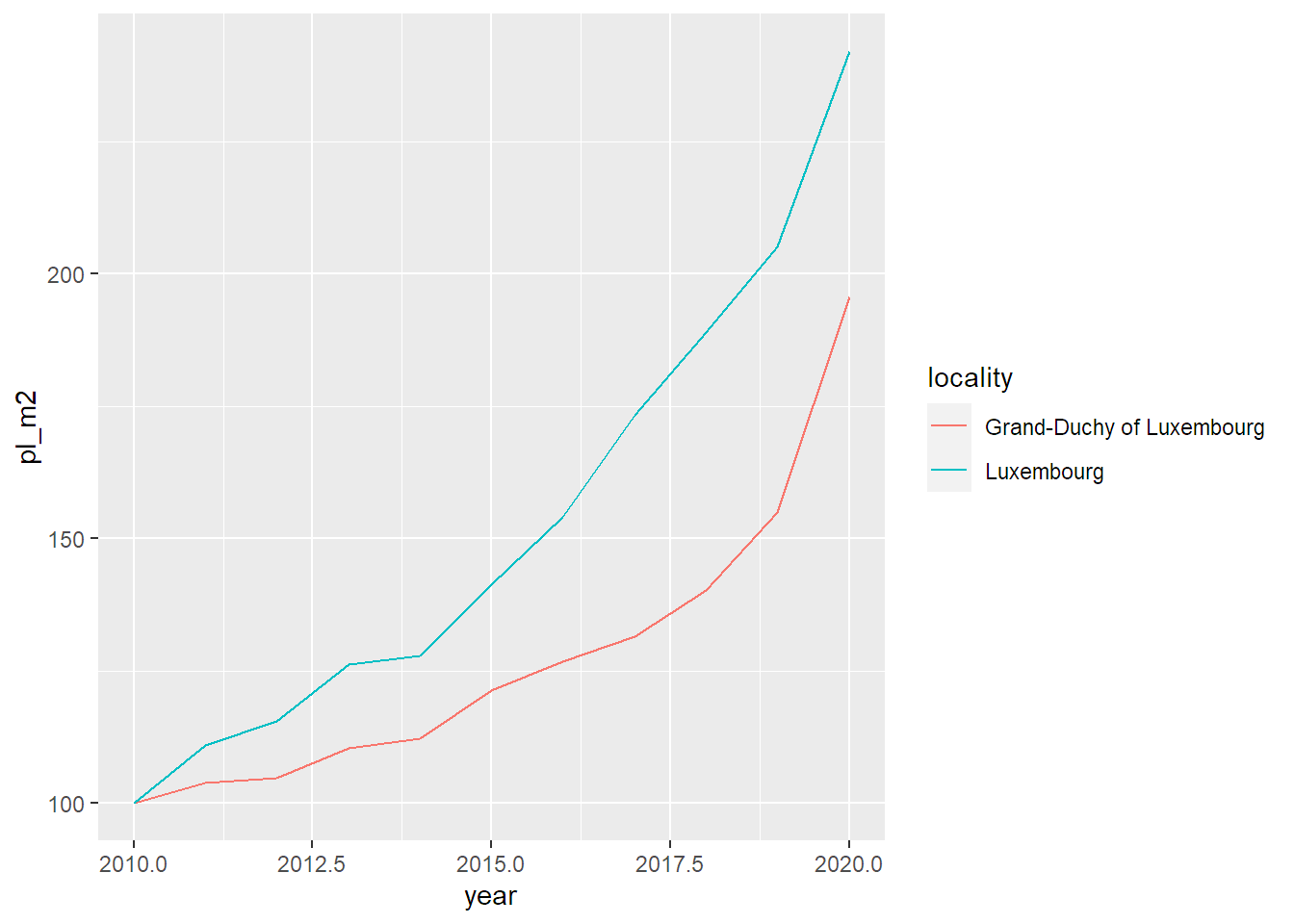## Plot for commune: Esch-sur-Alzette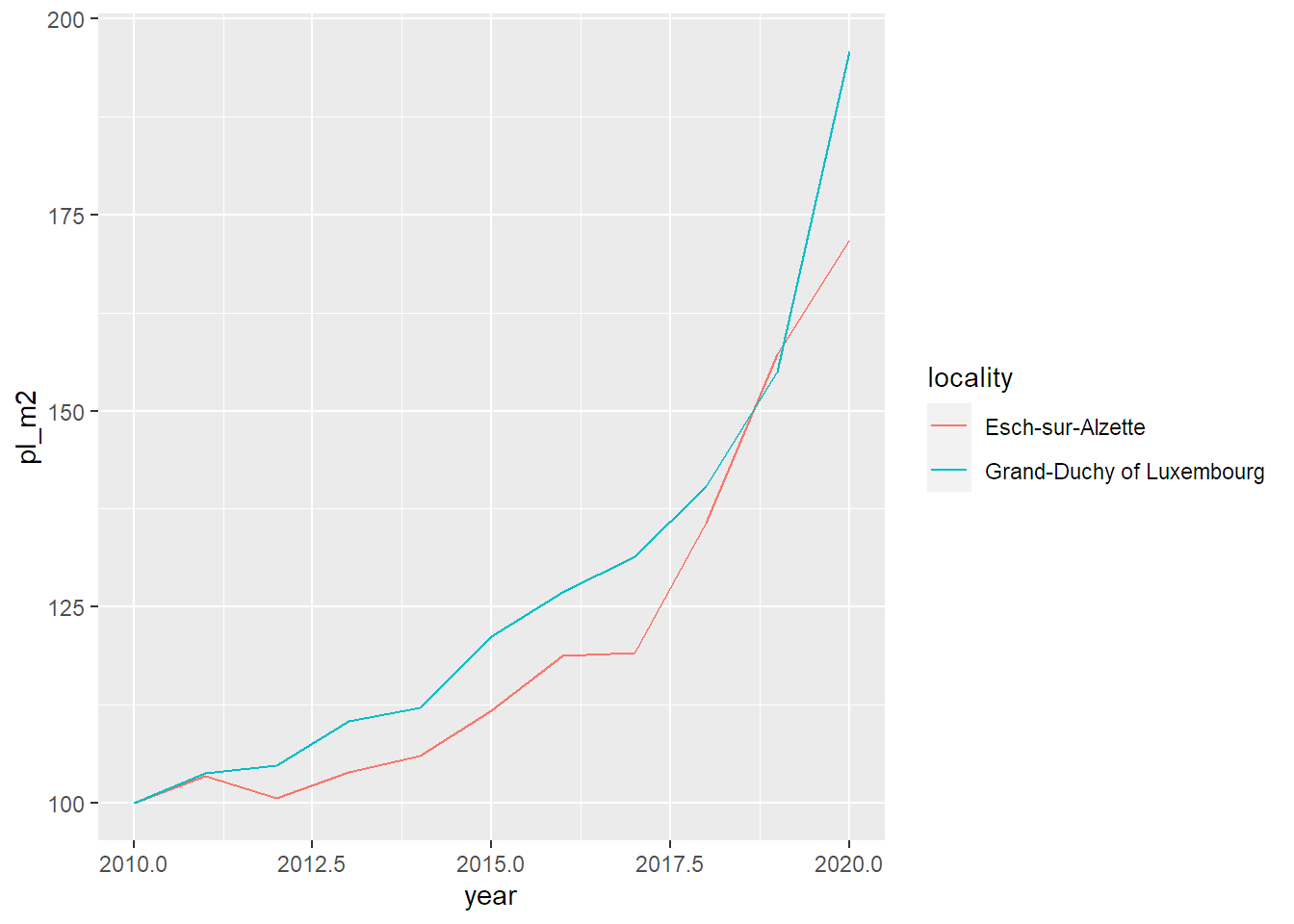## Plot for commune: Mamer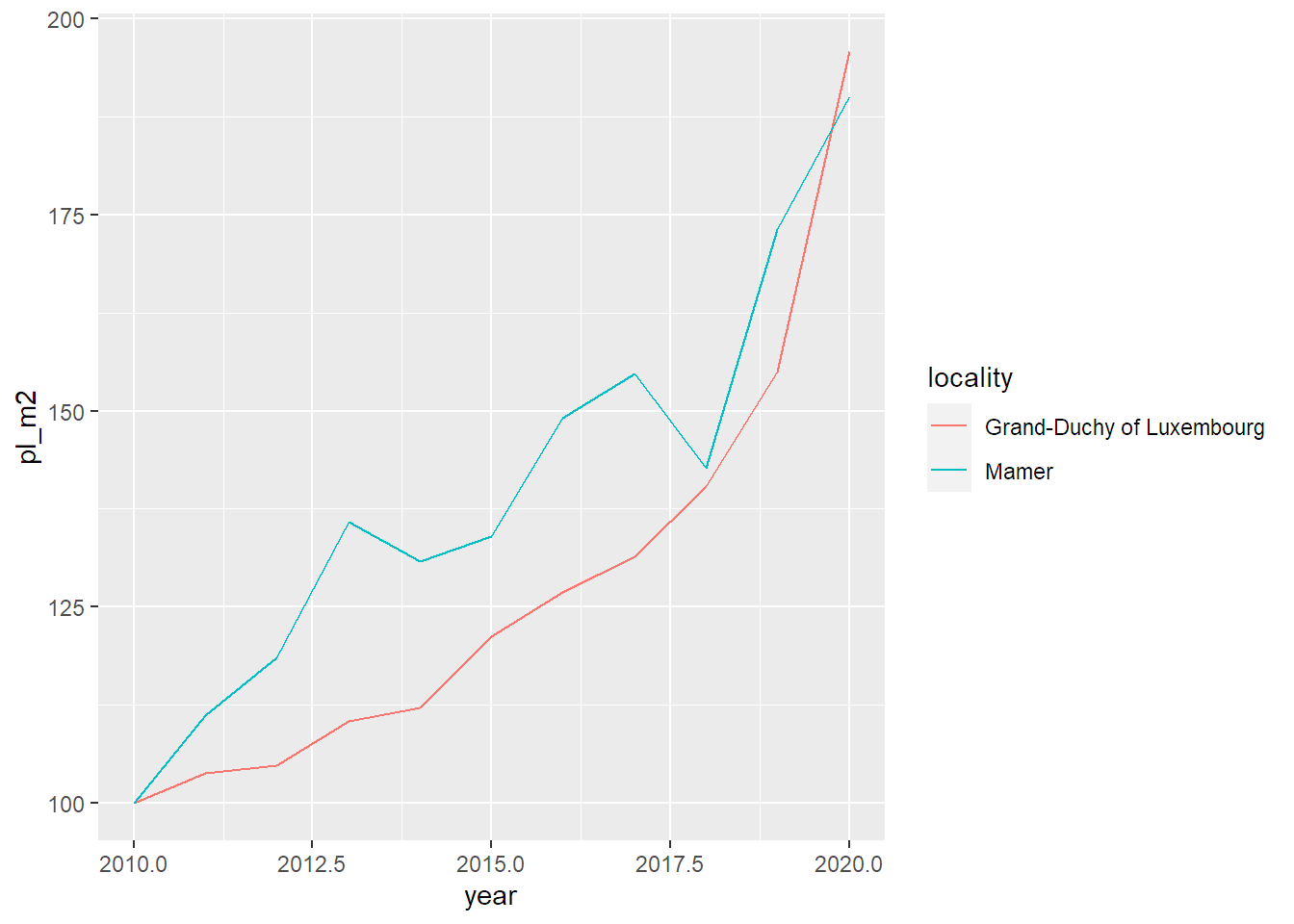## Plot for commune: Schengen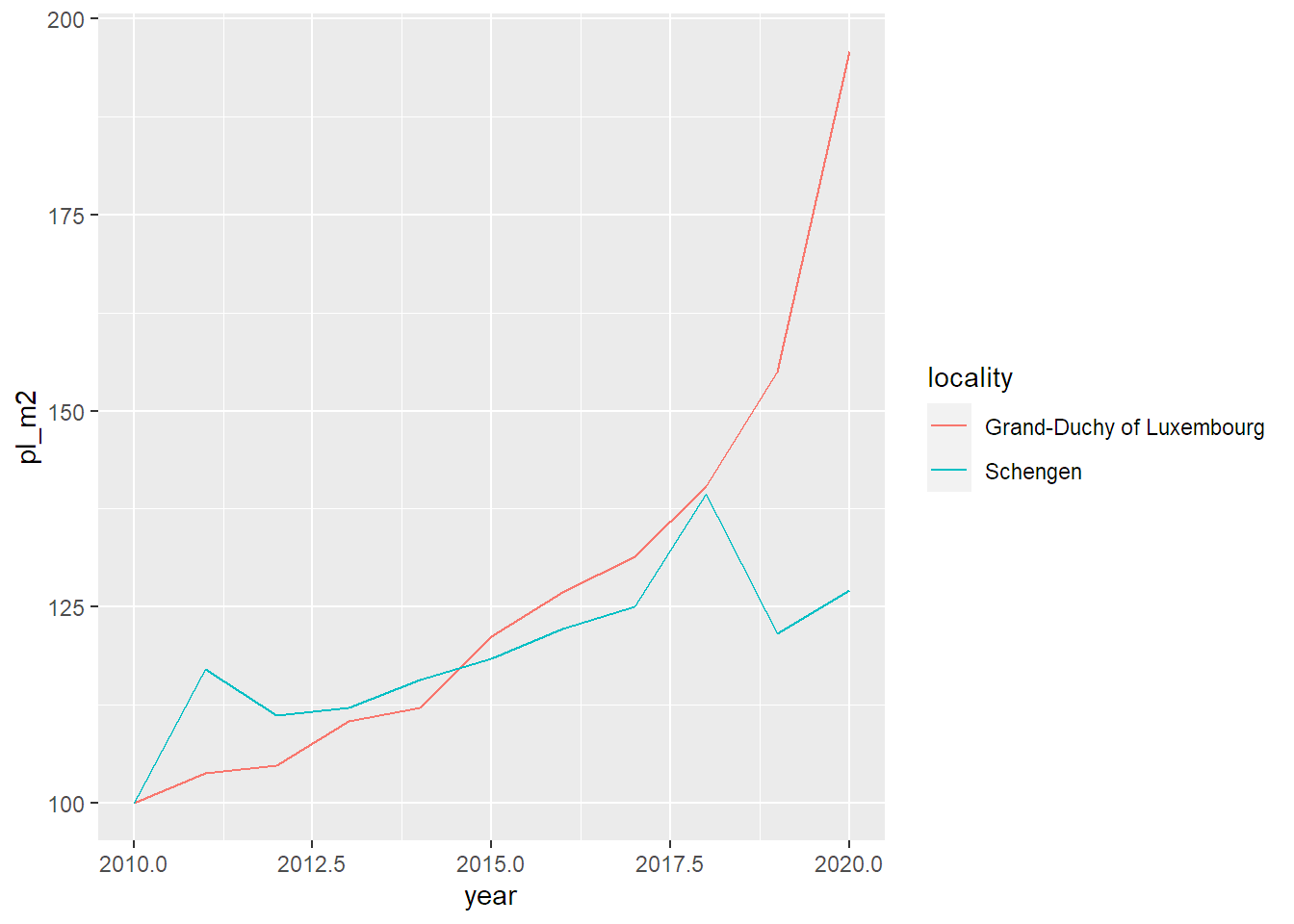## Plot for commune: Wincrange